# Triangular Pyramid

A triangular pyramid is a geometric shape that has a triangular base and three triangular faces. It has a vertex, common to all the three lateral faces of a triangular pyramid. If all the three triangular faces are equilateral, then such a pyramid is called a tetrahedron.

A Pyramid is a polyhedron that has a base and three or more than three triangular faces that meeting at a point above the base (the apex). Triangular pyramids are formed solely from triangles. The 3 triangular sides slant upwards to form the triangular base. As it is formed from four triangles, a triangular-based pyramid is also called a tetrahedron. Learn more about pyramids here.

The base of the pyramid could be based on different types of triangles. If all the faces are equilateral triangles or triangles whose edges are all equal lengths, the pyramid is called a regular tetrahedron. If the triangles have edges of various lengths, the pyramid is an irregular tetrahedron.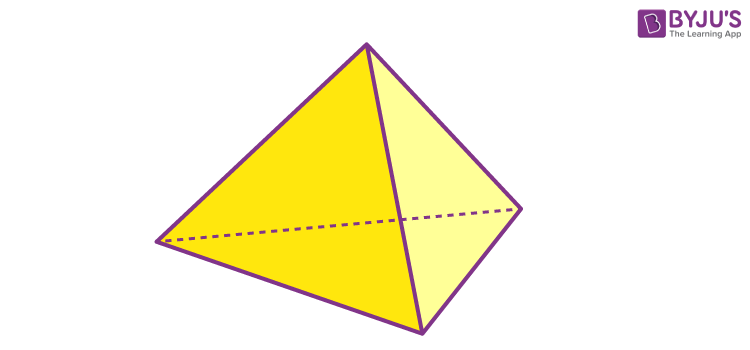## Triangular Pyramid Definition

A triangular pyramid is a pyramid which has a triangular base. In geometry, vertices are essentially corners. All triangular-based pyramids, either regular or irregular, have four vertices.

Triangular-based pyramids have 6 edges, 3 are along the base and 3 are extending up from the base. If the six edges are of the same length, all the triangles are equilateral, and the pyramid is called a regular tetrahedron.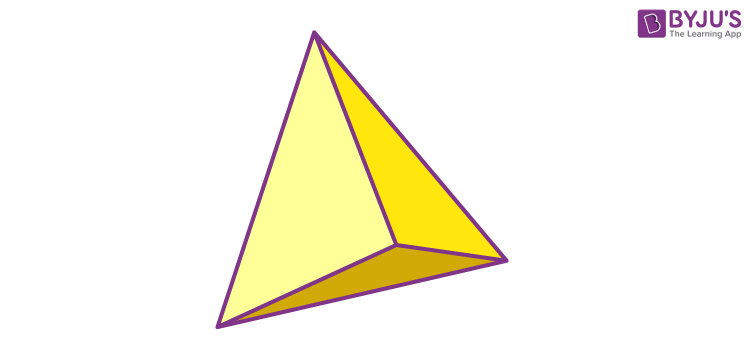## Types of Triangular Pyramids

The different types of triangular pyramids are :

• Regular Triangular Pyramid
• Non-regular Pyramid
• Right Triangular Pyramid

### Regular Triangular Pyramid

A pyramid has a base with sides that are equal in length. For the triangular pyramid, the base of regular tetrahedron should be an equilateral triangle.

### Non-Regular Triangular Pyramid

A pyramid has a base with sides that have different lengths. The base of the triangular pyramid has unequal sides. Or we can say, the base could be a scalene triangle or an isosceles triangle.

### Right Triangular Pyramid

A three-Dimensional shape with a right-angle triangle at its base extruding up to a single point is a right-triangular pyramid.

## Properties of Triangular Pyramid

• The triangular pyramid has 4 faces
• The 3 side faces of the triangular pyramid are triangles
• The base is also triangular in shape
• It has 4 vertices (corner points)
• It has 6 edges
• Triangular pyramid can be regular, irregular and right-angled
• A regular triangular pyramid has equilateral triangles for all four faces

## Triangular Pyramid Formula

The formula for the area and volume of the triangular pyramid is given here. We can use these formulas to solve the problems based on them.

### Volume of Triangular Pyramid

To find the volume of a triangular-based pyramid, multiply the area of the triangular base and the height of the pyramid (measured from the base to the apex). Then, divide this number by three.

Volume = ⅓ × Base Area × Height

### Surface Area of Triangular Pyramid

To determine the surface area of a triangular-based pyramid, add together the area of the base and the area of all the sides.

For regular tetrahedra, this calculation is simple. Find the length of the base and the height of one of the triangles. Multiply those measurements collectively and divide this number by two. This is the area of one of the triangles.

Now, multiply this area by four to account for all the triangular faces of the pyramid. For irregular tetrahedra, find the area of every triangle individually, using the area formula. Then, add all the areas together.

Surface Area = (Base area) + ½ × Perimeter × (Slant length)

## Net of a Triangular Pyramid

A net of the triangular pyramid is the pattern formed when the surface of it is laid out flat showing each triangular face of the figure. For different types of solids, the net pattern is different.

To find the net of a solid we should keep a note of the following points:

• Make sure the pyramid and the net have an equal number of faces.
• The shapes of the faces of the pyramid should match the shapes of the corresponding faces in the net.
• Imagine how the net can be folded to form the pyramid and assure that all the sides fit together properly.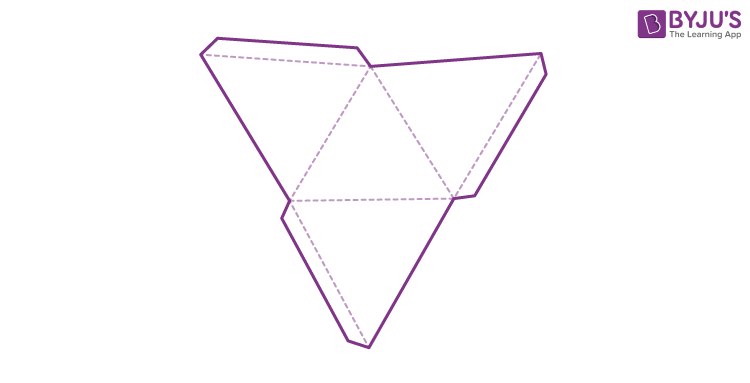Note: Nets are helpful when we need to find the surface area of the solids.

## Triangular Pyramid Solved Examples

Example 1: Find the surface area of a triangular pyramid with the base area is 28cm.sq., the perimeter is 20cm, slant length is 5cm.

Solution:

Surface area = [Base area] + ½ × Perimeter × [Slant length]

= 28 + ½ × 20 × 5

= 28 + 50

= 78 cm.sq.

Example 2: Find the volume of a triangular pyramid with a base area is 28cm, height is 4.5cm.

Solution:

Volume = ⅓ × Base Area × Height

= ⅓ × 28 × 4.5

= ⅓ × 126

= 42 cubic.cm.

Example 3: Find the volume of the following triangular pyramid, rounding your answer to two decimal places.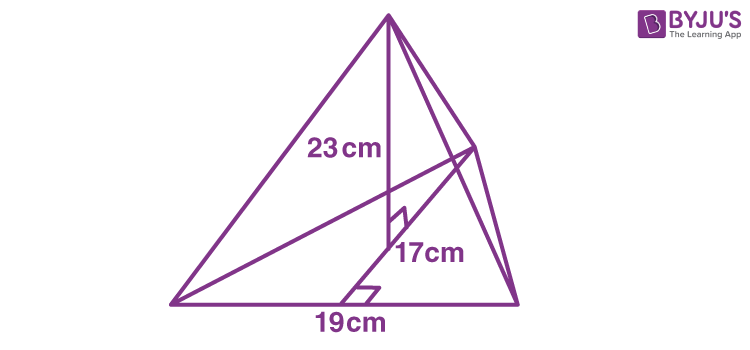Solution: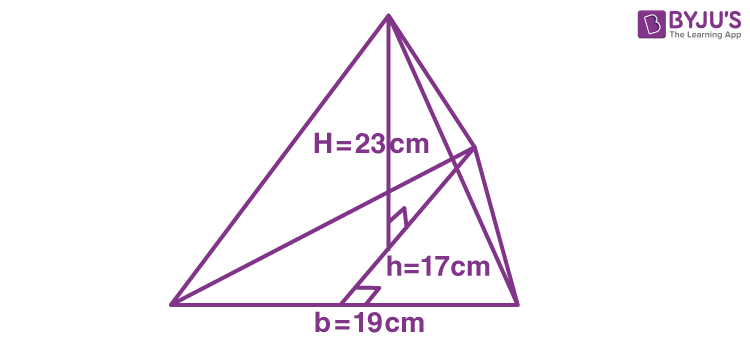V = ⅓ × AH

= ⅓ × (½ bh) H

= ⅙ bhH

= ⅙ × 19 × 17 × 23

= 1238.17 (Correct to 2 decimal places)

So, the volume is 1238.17 cm3

## Practise Questions

1. What is the volume of a regular triangular pyramid whose edge length is equal to 10cm?
2. Find the total surface area of a regular triangular pyramid whose each edge is equal to 5 cm.

## Frequently Asked Questions – FAQs

Q1

### What is a triangular pyramid?

A triangular pyramid is a geometric shape that has a triangular base and three triangular faces, having a common vertex.
Q2

### How many faces does a triangular pyramid have?

A triangular pyramid has four faces, one in the base and three lateral faces.
Q3

### How many vertices does a triangular pyramid have?

A triangular pyramid has four vertices. One vertex is common to all three faces of the pyramid.
Q4

### What are the types of triangular pyramids?

There are three types of triangular pyramids. They are regular, irregular, and right-angled triangular pyramids.
Quiz on Triangular Pyramid